# [长文]从《100 Go Mistakes》我总结了什么？

``````    var client *http.Client
if tracing {
client, err := createClientWithTracing()
if err != nil {
return err
}
log.Println(client)
} else {
client, err := createDefaultClient()
if err != nil {
return err
}
log.Println(client)
}``````

``````    var client *http.Client
var err error
if tracing {
client, err = createClientWithTracing()
} else {
...
}
if err != nil { // 防止重复代码
return err
}``````

``go install golang.org/x/tools/go/analysis/passes/shadow/cmd/shadow``

`````` go vet -vettool=C:\Users\luozhiyun\go\bin\shadow.exe .\main.go
# command-line-arguments
.\main.go:15:3: declaration of "client" shadows declaration at line 13
.\main.go:21:3: declaration of "client" shadows declaration at line 13``````

## 慎用 init 函数

### init 函数会在全局变量之后被执行

init 函数并不是最先被执行的，如果声明了 const 或 全局变量，那么init 函数会在它们之后执行：

``````package main

import "fmt"

var a = func() int {
fmt.Println("a")
return 0
}()

func init() {
fmt.Println("init")
}

func main() {
fmt.Println("main")
}

// output
a
init
main``````

### init初始化按解析的依赖关系顺序执行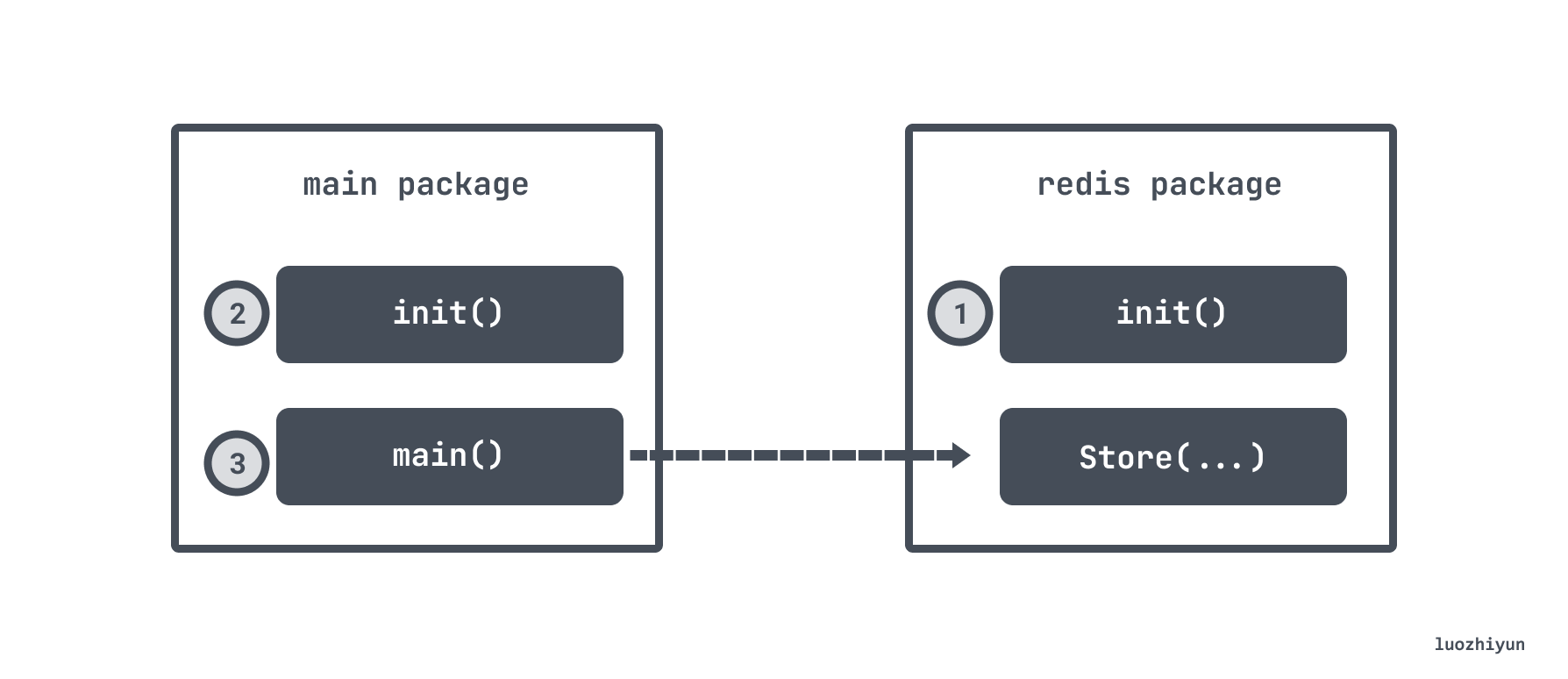### 扰乱单元测试

``````var db *sql.DB
func init(){
dataSourceName := os.Getenv("MYSQL_DATA_SOURCE_NAME")
d, err := sql.Open("mysql", dataSourceName)
if err != nil {
log.Panic(err)
}
db = d
}``````

## embed types优缺点

embed types 指的是我们在 struct 里面定义的匿名的字段，如：

``````type Foo struct {
Bar
}
type Bar struct {
Baz int
}``````

``````type Logger struct {
writeCloser io.WriteCloser
}

func (l Logger) Write(p []byte) (int, error) {
return l.writeCloser.Write(p)
}

func (l Logger) Close() error {
return l.writeCloser.Close()
}

func main() {
l := Logger{writeCloser: os.Stdout}
_, _ = l.Write([]byte("foo"))
_ = l.Close()
}``````

``````type Logger struct {
io.WriteCloser
}

func main() {
l := Logger{WriteCloser: os.Stdout}
_, _ = l.Write([]byte("foo"))
_ = l.Close()
}``````

``````type InMem struct {
sync.Mutex
m map[string]int
}

func New() *InMem {
return &InMem{m: make(map[string]int)}
}``````

Mutex 一般并不想 export， 只想在 InMem 自己的函数中使用，如：

``````func (i *InMem) Get(key string) (int, bool) {
i.Lock()
v, contains := i.m[key]
i.Unlock()
return v, contains
}``````

``````m := inmem.New()
m.Lock() // ??``````

## Functional Options Pattern传递参数

• 设置一个不导出的 struct 叫 options，用来存放配置参数；
• 创建一个类型 `type Option func(options *options) error`，用这个类型来作为返回值；

``````type options struct {
port *int
}

type Option func(options *options) error

func WithPort(port int) Option {
// 所有的类型校验，赋值，初始化啥的都可以放到这个闭包里面做
return func(options *options) error {
if port < 0 {
return errors.New("port should be positive")
}
options.port = &port
return nil
}
}``````

``````func NewServer(addr string, opts ...Option) (*http.Server, error) {
var options options
// 遍历所有的 Option
for _, opt := range opts {
// 执行闭包
err := opt(&options)
if err != nil {
return nil, err
}
}

// 接下来可以填充我们的业务逻辑，比如这里设置默认的port 等等
var port int
if options.port == nil {
port = defaultHTTPPort
} else {
if *options.port == 0 {
port = randomPort()
} else {
port = *options.port
}
}

// ...
}``````

``````server, err := httplib.NewServer("localhost",
httplib.WithPort(8080),
httplib.WithTimeout(time.Second)) ``````

``server, err := httplib.NewServer("localhost") ``

## 小心八进制整数

``````    sum := 100 + 010
fmt.Println(sum)``````

``    file, err := os.OpenFile("foo", os.O_RDONLY, 0644)``

``    file, err := os.OpenFile("foo", os.O_RDONLY, 0o644)``

## float 的精度问题

1. 符号位(Sign) : 0代表正，1代表为负

2. 指数位（Exponent）:用于存储科学计数法中的指数数据，并且采用移位存储

3. 尾数部分（Mantissa）：尾数部分``````func f1(n int) float64 {
result := 10_000.
for i := 0; i < n; i++ {
result += 1.0001
}
return result
}

func f2(n int) float64 {
result := 0.
for i := 0; i < n; i++ {
result += 1.0001
}
return result + 10_000.
}``````

n Exact result `f1` `f2`
10 10010.001 10010.000999999993 10010.001
1k 11000.1 11000.099999999293 11000.099999999982
1m 1.0101e+06 1.0100999999761417e+06 1.0100999999766762e+06

``````a := 100000.001
b := 1.0001
c := 1.0002

fmt.Println(a * (b + c))
fmt.Println(a*b + a*c)``````

``````200030.00200030004
200030.0020003``````

``````a := decimal.NewFromFloat(100000.001)
b := decimal.NewFromFloat(1.0001)
c := decimal.NewFromFloat(1.0002)

## slice 相关注意点

### 区分 slice 的 length 和 capacity

``s := make([]int, 3, 6)``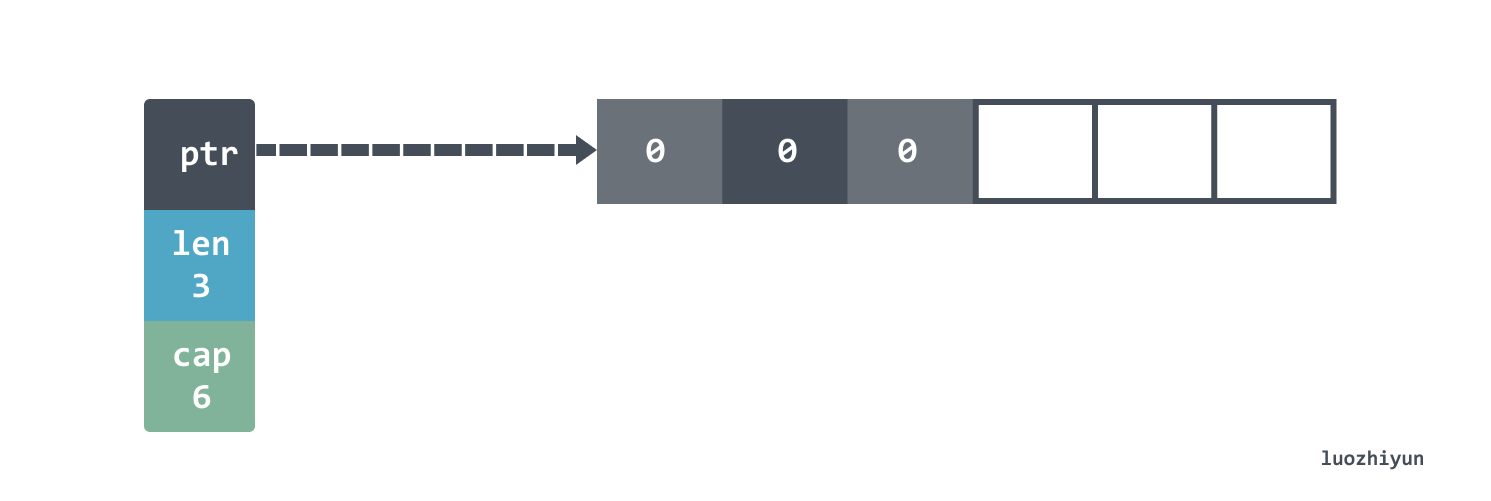slice 的底层实际上指向了一个数组。当然，由于我们的 length 是 3，所以这样设置 `s = 0` 会 panic 的。需要使用 append 才能添加新元素。

``panic: runtime error: index out of range  with length 3``

``````s1 := make([]int, 3, 6)
s2 := s1[1:3]``````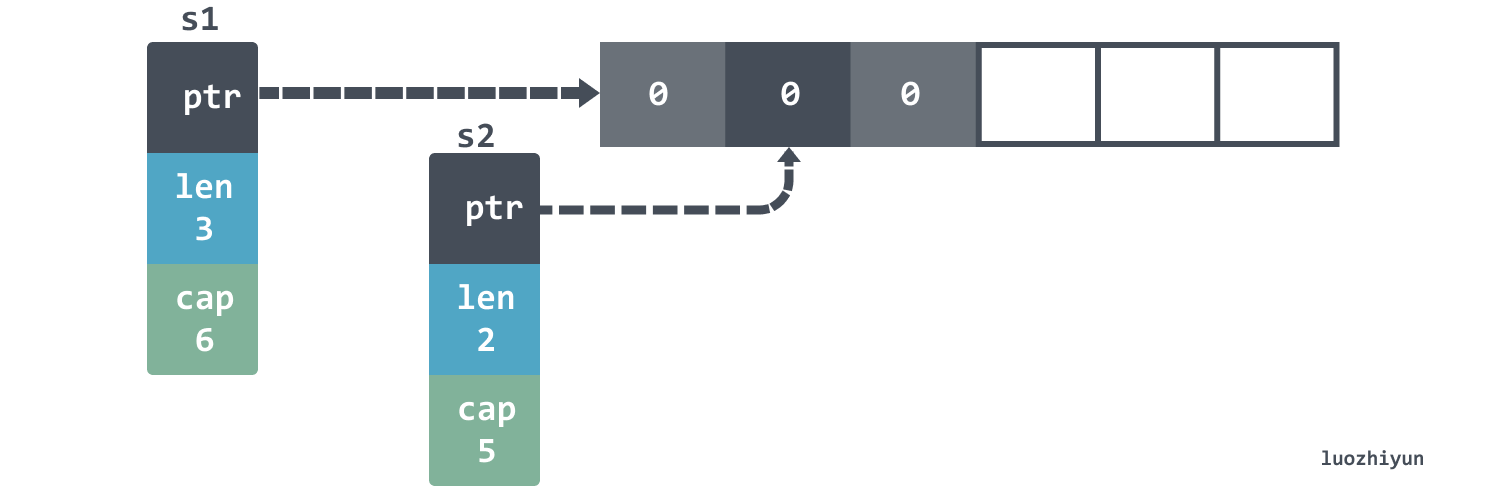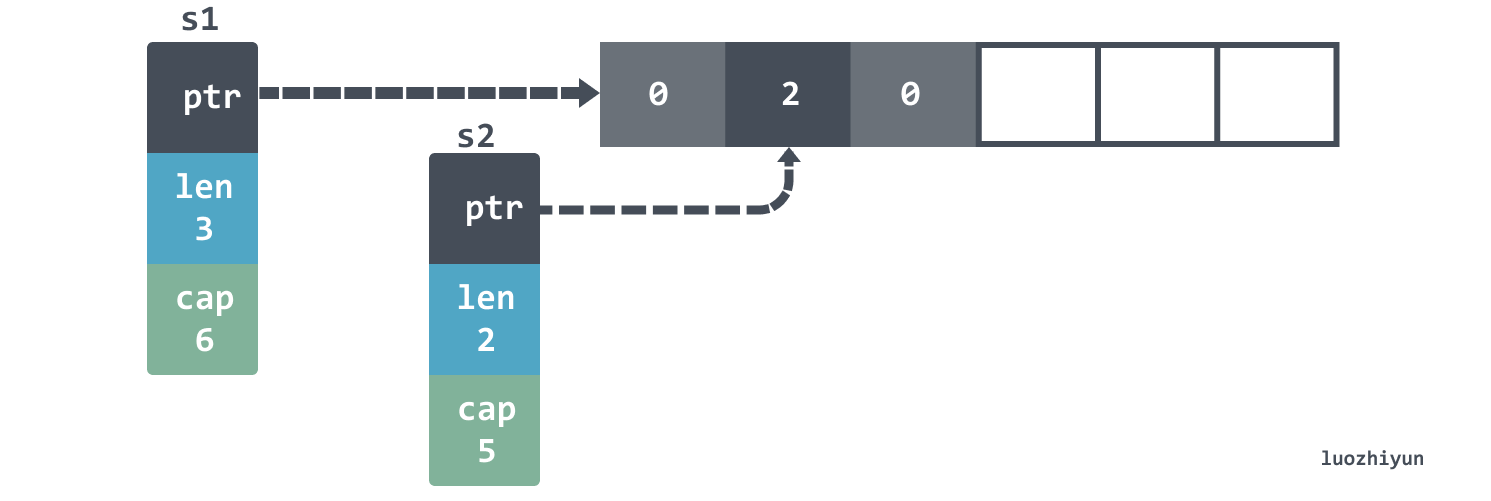``````s2 = append(s2, 3)

fmt.Println(s1) // [0 2 0]
fmt.Println(s2) // [2 0 3]``````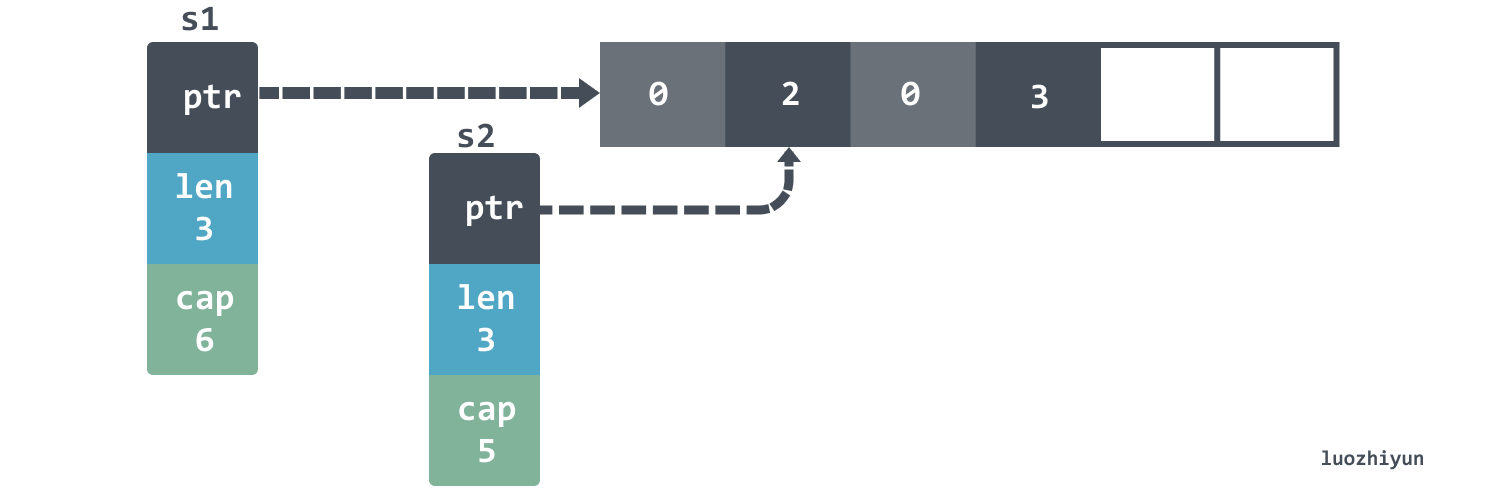s1 的 len 并没有被改变，所以看到的还是3元素。

``````    s1 = append(s1, 4)
fmt.Println(s1) // [0 2 0 4]
fmt.Println(s2) // [2 0 4]``````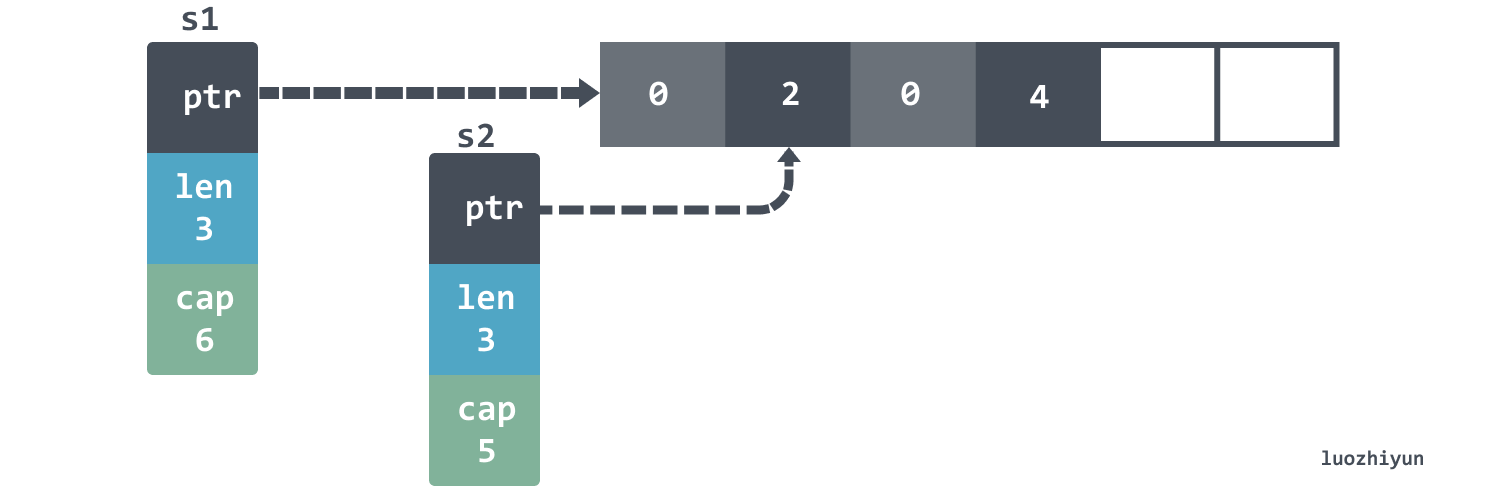``````s2 = append(s2, 5, 6, 7)
fmt.Println(s1) //[0 2 0 4]
fmt.Println(s2) //[2 0 4 5 6 7]``````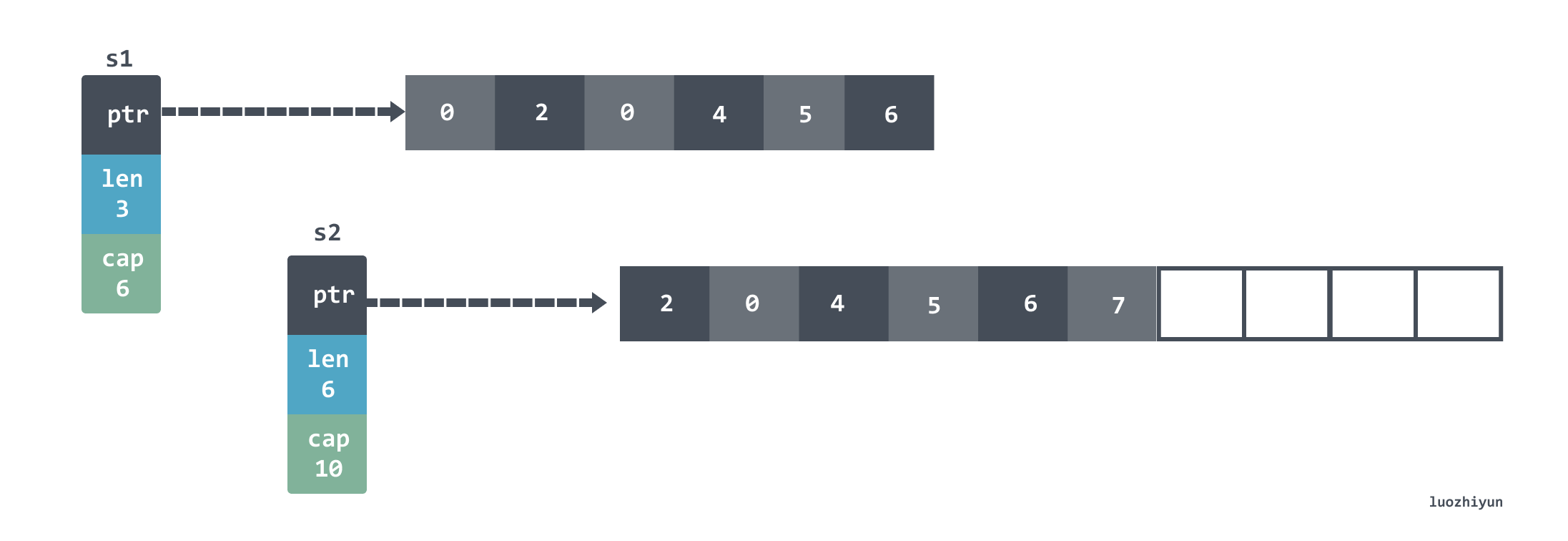``````s1 := []int{1, 2, 3}
s2 := s1[1:2]
s3 := append(s2, 10)``````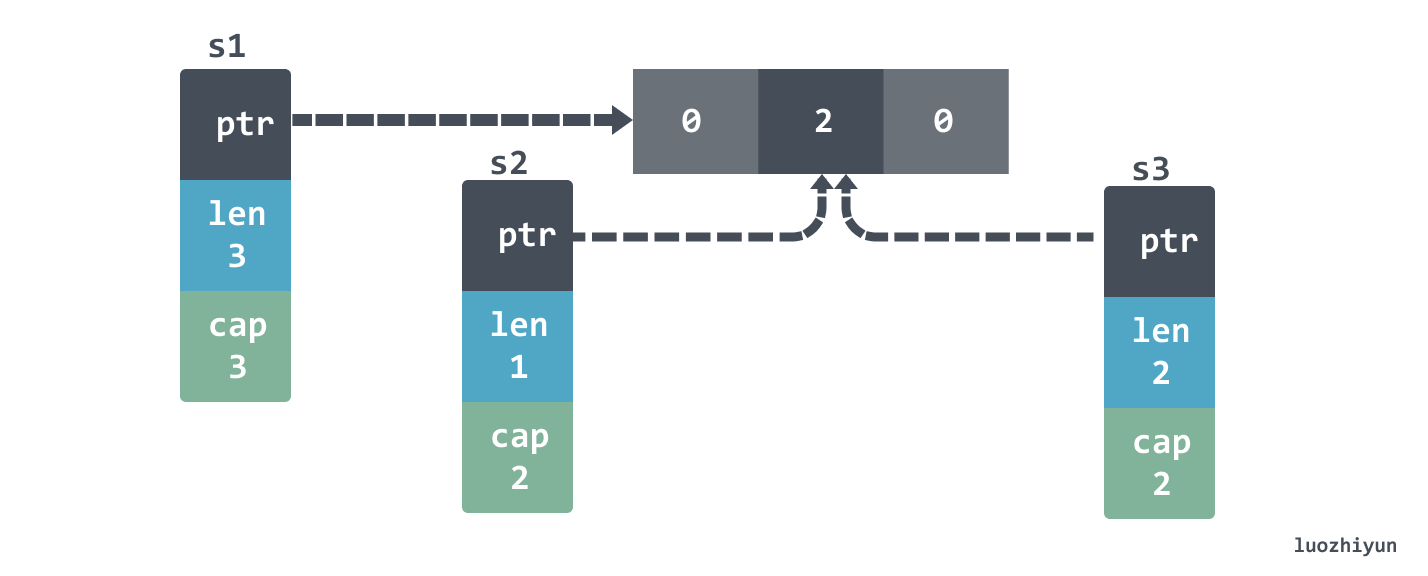``s1=[1 2 10], s2=, s3=[2 10]``

### slice 初始化

``````func main() {
var s []string
log(1, s)

s = []string(nil)
log(2, s)

s = []string{}
log(3, s)

s = make([]string, 0)
log(4, s)
}

func log(i int, s []string) {
fmt.Printf("%d: empty=%t\tnil=%t\n", i, len(s) == 0, s == nil)
}``````

``````1: empty=true   nil=true
2: empty=true   nil=true
3: empty=true   nil=false
4: empty=true   nil=false``````

``````func f() []string {
var s []string
if foo() {
s = append(s, "foo")
}
if bar() {
s = append(s, "bar")
}
return s
}``````

``s := []string{"foo", "bar", "baz"}``

``````src := []int{0, 1, 2}
dst := append([]int(nil), src...)``````

``````BenchmarkConvert_EmptySlice-4                22     49739882 ns/op
BenchmarkConvert_GivenCapacity-4             86     13438544 ns/op
BenchmarkConvert_GivenLength-4               91     12800411 ns/op``````

### copy slice

``````src := []int{0, 1, 2}
var dst []int
copy(dst, src)
fmt.Println(dst) // []``````

``````src := []int{0, 1, 2}
dst := make([]int, len(src))
copy(dst, src)
fmt.Println(dst) //[0 1 2]``````

``````src := []int{0, 1, 2}
dst := append([]int(nil), src...)``````

### slice capacity内存释放问题

``````type Foo struct {
v []byte
}

func keepFirstTwoElementsOnly(foos []Foo) []Foo {
return foos[:2]
}

func main() {
foos := make([]Foo, 1_000)
printAlloc()

for i := 0; i < len(foos); i++ {
foos[i] = Foo{
v: make([]byte, 1024*1024),
}
}
printAlloc()

two := keepFirstTwoElementsOnly(foos)
runtime.GC()
printAlloc()
runtime.KeepAlive(two)
}``````

``````func printAlloc() {
var m runtime.MemStats
fmt.Printf("%d KB\n", m.Alloc/1024)
}``````

``````387 KB
1024315 KB
1024319 KB``````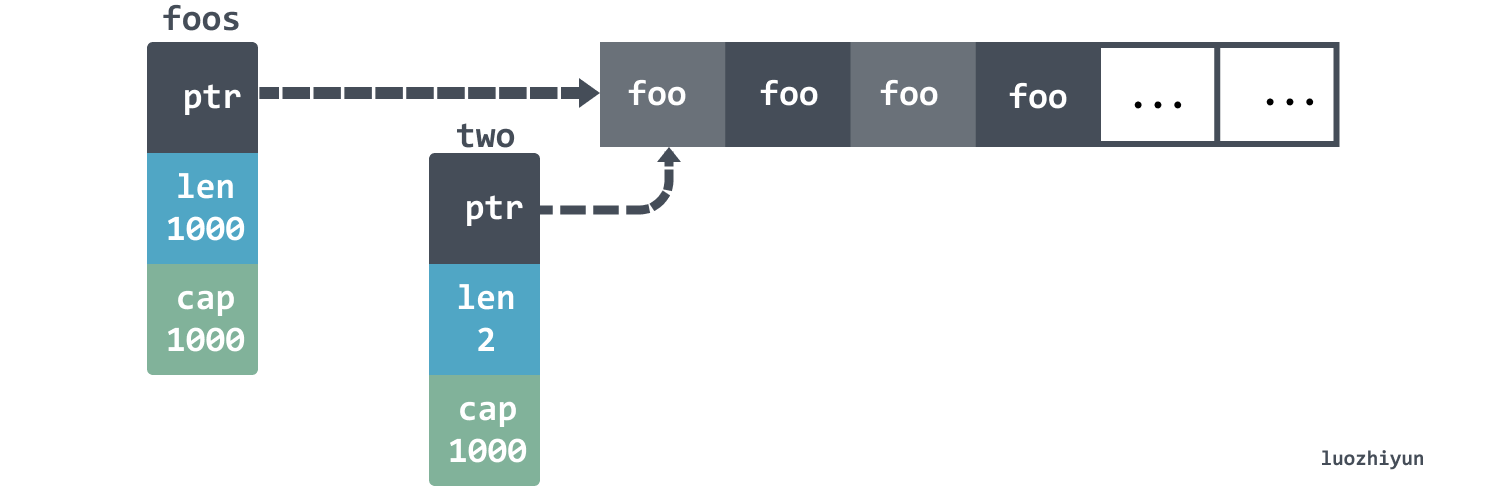``````func keepFirstTwoElementsOnly(foos []Foo) []Foo {
res := make([]Foo, 2)
copy(res, foos)
return res
}``````

``````func keepFirstTwoElementsOnly(foos []Foo) []Foo {
for i := 2; i < len(foos); i++ {
foos[i].v = nil
}
return foos[:2]
}``````

## 注意 range

### copy 的问题

``````type account struct {
balance float32
}

accounts := []account{
{balance: 100.},
{balance: 200.},
{balance: 300.},
}
for _, a := range accounts {
a.balance += 1000
} ``````

``[{100} {200} {300}]``

``````for i := range accounts {
accounts[i].balance += 1000
}``````

range slice 的话也会copy一份：

``````s := []int{0, 1, 2}
for range s {
s = append(s, 10)
} ``````

### 指针问题

``````type Customer struct {
ID      string
Balance float64
}

test := []Customer{
{ID: "1", Balance: 10},
{ID: "2", Balance: -10},
{ID: "3", Balance: 0},
}

for _, customer := range test {
fmt.Printf("%p\n", &customer) //我们想要获取这个指针的时候
} ``````

``````0x1400000e240
0x1400000e240
0x1400000e240````````````    for _, customer := range test {
current := customer
fmt.Printf("%p\n", &current)
}``````

## 注意break作用域

``````for i := 0; i < 5; i++ {
fmt.Printf("%d ", i)

switch i {
default:
case 2:
break
}
} ``````

``````loop:
for i := 0; i < 5; i++ {
fmt.Printf("%d ", i)
switch i {
default:
case 2:
break loop
}
}``````

``````    for {
select {
case <-ch:
// Do something
case <-ctx.Done():
break
}
}``````

``````    loop:
for {
select {
case <-ch:
// Do something
case <-ctx.Done():
break loop
}
}``````

## defer

### 注意defer的调用时机

``````func readFiles(ch <-chan string) error {
for path := range ch {
file, err := os.Open(path)
if err != nil {
return err
}

defer file.Close()

// Do something with file
}
return nil
} ``````

``````func readFiles(ch <-chan string) error {
for path := range ch {
if err := readFile(path); err != nil {
return err
}
}
return nil
}

file, err := os.Open(path)
if err != nil {
return err
}

defer file.Close()

// Do something with file
return nil
} ``````

### 注意defer的参数

defer 声明时会先计算确定参数的值。

``````func a() {
i := 0
defer notice(i) // 0
i++
return
}

func notice(i int) {
fmt.Println(i)
}``````

``````func a() {
i := 0
defer notice(&i) // 0
i++
return
}``````

### defer下的闭包

``````func a() int {
i := 0
defer func() {
fmt.Println(i + 1) //12
}()
i++
return i+10
}

func TestA(t *testing.T) {
fmt.Println(a()) //11
}``````

``先计算（i+10）-> (call defer) -> (return)``

## string相关

### 迭代带来的问题

``````    s := "hêllo"
for i := range s {
fmt.Printf("position %d: %c\n", i, s[i])
}
fmt.Printf("len=%d\n", len(s))``````

``````position 0: h
position 1: Ã
position 3: l
position 4: l
position 5: o
len=6``````

s h ê l l o
[]byte(s) 68 c3 aa 6c 6c 6f

``````    s := "hêllo"
for i, v := range s {
fmt.Printf("position %d: %c\n", i, v)
}``````

``````    s := "hêllo"
runes := []rune(s)
for i, _ := range runes {
fmt.Printf("position %d: %c\n", i, runes[i])
}``````

``````position 0: h
position 1: ê
position 2: l
position 3: l
position 4: o``````

### 截断带来的问题

``````func (s store) handleLog(log string) error {
if len(log) < 36 {
return errors.New("log is not correctly formatted")
}
uuid := log[:36]
s.store(uuid)
// Do something
} ``````

``````func (s store) handleLog(log string) error {
if len(log) < 36 {
return errors.New("log is not correctly formatted")
}
uuid := strings.Clone(log[:36])  // copy一份
s.store(uuid)
// Do something
} ``````

## interface 类型返回的非nil问题

``````type MultiError struct {
errs []string
}

func (m *MultiError) Add(err error) {
m.errs = append(m.errs, err.Error())
}

func (m *MultiError) Error() string {
return strings.Join(m.errs, ";")
}``````

``````func Validate(age int, name string) error {
var m *MultiError
if age < 0 {
m = &MultiError{}
}
if name == "" {
if m == nil {
m = &MultiError{}
}
}

return m
}

func Test(t *testing.T) {
if err := Validate(10, "a"); err != nil {
t.Errorf("invalid")
}
}``````

``invalid <nil>``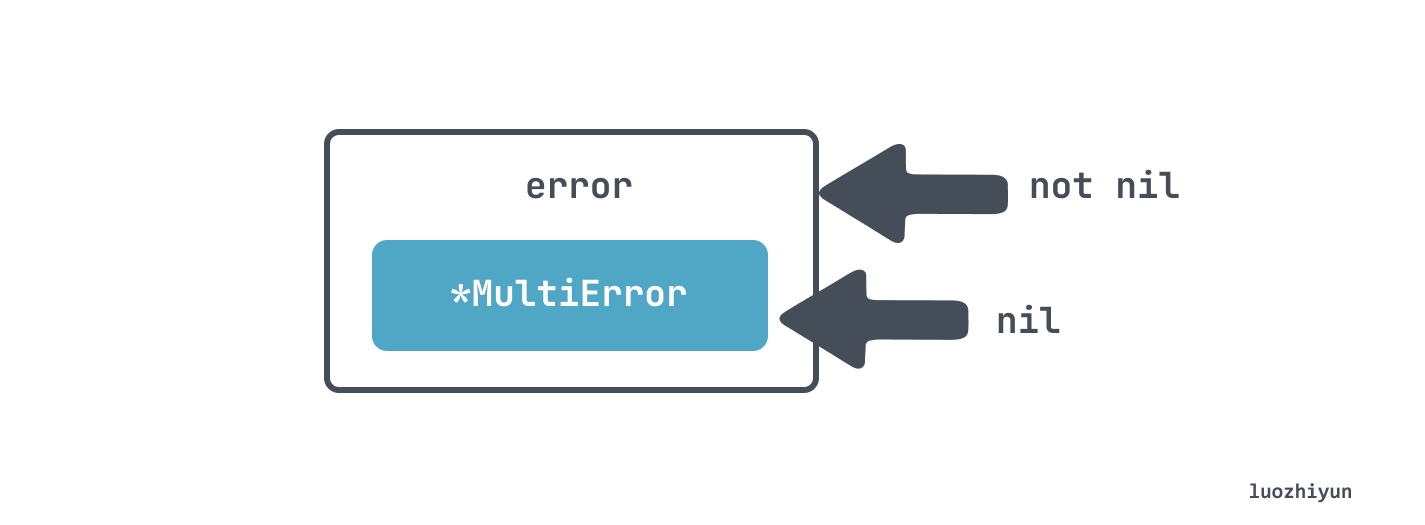## Error

### error wrap

`````` err:= xxx()
if err != nil {
return err
}``````

`````` err:= xxx()
if err != nil {
return XXError{Err: err}
}``````

`````` if err != nil {
return fmt.Errorf("xxx failed: %w", err)
}``````

`````` if err != nil {
return fmt.Errorf("xxx failed: %v", err)
}``````

### error Is & As

``````var BaseErr = errors.New("base error")

func main() {
err1 := fmt.Errorf("wrap base: %w", BaseErr)
err2 := fmt.Errorf("wrap err1: %w", err1)
println(err2 == BaseErr)

if !errors.Is(err2, BaseErr) {
panic("err2 is not BaseErr")
}
println("err2 is BaseErr")
} ``````

``````false
err2 is BaseErr``````

`errors.As`主要用来做类型判断，原因也是和上面一样，error 被 wrap 之后我们通过 `err.(type)`无法直接判断，`errors.As`会用 `Unwrap` 方法拆包装，然后挨个判断类型。使用如下：

``````type TypicalErr struct {
e string
}

func (t TypicalErr) Error() string {
return t.e
}

func main() {
err := TypicalErr{"typical error"}
err1 := fmt.Errorf("wrap err: %w", err)
err2 := fmt.Errorf("wrap err1: %w", err1)
var e TypicalErr
if !errors.As(err2, &e) {
panic("TypicalErr is not on the chain of err2")
}
println("TypicalErr is on the chain of err2")
println(err == e)
} ``````

``````TypicalErr is on the chain of err2
true``````

### 处理defer中的error

``````    func getBalance(db *sql.DB, clientID string) (
float32, error) {
rows, err := db.Query(query, clientID)
if err != nil {
return 0, err
}
defer rows.Close()

// Use rows
}``````

``````defer func() {
err := rows.Close()
if err != nil {
log.Printf("failed to close rows: %v", err)
}
return err //无法通过编译
}()``````

``````    func getBalance(db *sql.DB, clientID string) (balance float32, err error) {
rows, err = db.Query(query, clientID)
if err != nil {
return 0, err
}
defer func() {
err = rows.Close()
}()

// Use rows
}``````

``````defer func() {
closeErr := rows.Close()
if err != nil {
if closeErr != nil {
log.Printf("failed to close rows: %v", err)
}
return
}
err = closeErr
}()``````

## happens before保证

1. 创建 goroutine 发生先于 goroutine 执行，所以下面这段代码先读一个变量，然后在 goroutine 中写变量不会发生data race问题：

``````i := 0
go func() {
i++
}()``````
2. goroutine 退出没有任何 happen before保证，例如下面代码会有 data race ：

``````i := 0
go func() {
i++
}()
fmt.Println(i)``````
3. channel 操作中 send 操作是 happens before receive 操作 ：

``````var c = make(chan int, 10)
var a string

func f() {
a = "hello, world"
c <- 0
}

func main() {
go f()
<-c
print(a)
}``````

上面执行顺序应该是：

``variable change -》channel send -》channel receive -》variable read``

上面能够保证一定输出 `"hello, world"`

4. close channel 是 happens before receive 操作，所以下面这个例子中也不会有data race问题：

``````i := 0
ch := make(chan struct{})
go func() {
<-ch
fmt.Println(i)
}()
i++
close(ch)``````
5. 在无缓冲的 channel 中 receive 操作是happens before send操作的，例如：

``````var c = make(chan int)
var a string

func f() {
a = "hello, world"
<-c
}

func main() {
go f()
c <- 0
print(a)
}``````

这里同样能保证输出 `hello, world`

## Context Values

``ctx := context.WithValue(parentCtx, "key", "value")``

`context.WithValue`是从 parentCtx 创建，所以创建出来的 ctx 既包含了父类的上下文信息，也包含了当前新加的上下文。

``fmt.Println(ctx.Value("key"))``

``````    package provider

type key string

const myCustomKey key = "key"

func f(ctx context.Context) {
ctx = context.WithValue(ctx, myCustomKey, "foo")
// ...
}``````

## 应多关注goroutine何时停止

``````ch := foo()
go func() {
for v := range ch {
// ...
}
}()``````

``````func main() {
newWatcher()

// Run the application
}

type watcher struct { /* Some resources */ }

func newWatcher() {
w := watcher{}
go w.watch()
}``````

``````func main() {
w := newWatcher()
defer w.close()

// Run the application
}

func newWatcher() watcher {
w := watcher{}
go w.watch()
return w
}

func (w watcher) close() {
// Close the resources
}``````

## Channel

### select & channel

select 和 channel 搭配起来往往有意想不到的效果，比如下面：

``````for {
select {
case v := <-messageCh:
fmt.Println(v)
case <-disconnectCh:
fmt.Println("disconnection, return")
return
}
} ``````

``````for i := 0; i < 10; i++ {
messageCh <- i
}
disconnectCh <- struct{}{}``````

``````0
1
2
3
4
disconnection, return``````

1. 使用无缓冲的 messageCh channel，这样在发送数据的时候会一直等待，直到数据被消费了才会往下走，相当于是个同步模型了；
2. 在 select 里面使用单个channel，比如面的 demo 中我们可以定义一种特殊的 tag 来结束 channel，当读到这个特殊的 tag 的时候 return，这样就没必要用两个 channel 了。

``````for {
select {
case v := <-messageCh:
fmt.Println(v)
case <-disconnectCh:
for {
select {
case v := <-messageCh:
fmt.Println(v)
default:
fmt.Println("disconnection, return")
return
}
}
}
}``````

### 不要使用nil channel

``````var ch chan int
ch <- 0 //block``````

``````var ch chan int
<-ch //block``````

### Channel 的 close 问题

channel 在 close 之后仍然可以接收数据的，例如：

``````    ch1 := make(chan int, 1)
close(ch1)
for {
v := <-ch1
fmt.Println(v)
}``````

``````func merge(ch1, ch2 <-chan int) <-chan int {
ch := make(chan int, 1)
go func() {
for {
select {
case v:= <-ch1:
ch <- v
case v:= <-ch2:
ch <- v
}
}
close(ch) // 永远运行不到
}()
return ch
}``````

``````    v, open := <-ch1
fmt.Print(v, open) //open返回false 表示没有被关闭``````

``````func merge(ch1, ch2 <-chan int) <-chan int {
ch := make(chan int, 1)
ch1Closed := false
ch2Closed := false

go func() {
for {
select {
case v, open := <-ch1:
if !open { // 如果已经关闭
ch1Closed = true //标记为true
break
}
ch <- v
case v, open := <-ch2:
if !open { // 如果已经关闭
ch2Closed = true//标记为true
break
}
ch <- v
}

if ch1Closed && ch2Closed {//都关闭了
close(ch)//关闭ch
return
}
}
}()
return ch
}``````

``````type Customer struct {
mutex sync.RWMutex
id    string
age   int
}

func (c *Customer) UpdateAge(age int) error {
c.mutex.Lock()
defer c.mutex.Unlock()

if age < 0 {
return fmt.Errorf("age should be positive for customer %v", c)
}

c.age = age
return nil
}

func (c *Customer) String() string {
fmt.Println("enter string method")
c.mutex.RLock()
defer c.mutex.RUnlock()
return fmt.Sprintf("id %s, age %d", c.id, c.age)
}``````

``mutex.Lock -> check age -> Format error -> call String() -> mutex.RLock``

## 错误使用 sync.WaitGroup

`sync.WaitGroup`通常用在并发中等待 goroutines 任务完成，用 Add 方法添加计数器，当任务完成后需要调用 Done 方法让计数器减一。等待的线程会调用 Wait 方法等待，直到`sync.WaitGroup` 内计数器为零。

``````wg := sync.WaitGroup{}
var v uint64

for i := 0; i < 3; i++ {
go func() {
wg.Done()
}()
}

wg.Wait()
fmt.Println(v)``````

## 不要拷贝 sync 类型

sync 包里面提供一些并发操作的类型，如 mutex、condition、wait gorup 等等，这些类型都不应该被拷贝之后使用。

``````type Counter struct {
mu       sync.Mutex
counters map[string]int
}

func (c Counter) Increment(name string) {
c.mu.Lock()
defer c.mu.Unlock()
c.counters[name]++
}

func NewCounter() Counter {
return Counter{counters: map[string]int{}}
}

func main() {
counter := NewCounter()
go counter.Increment("aa")
go counter.Increment("bb")
}``````

receiver 是一个值类型，所以调用 Increment 方法的时候实际上拷贝了一份 Counter 里面的变量。这里我们可以将 receiver 改成一个指针，或者将 `sync.Mutex` 变量改成指针类型。

2. 函数参数是 sync 包类型；
3. 函数参数的结构体里面包含了 sync 包类型；

``````» go vet .                                                                      bear@BEARLUO-MB7
# github.com/cch123/gogctuner/main
./main.go:53:9: Increment passes lock by value: github.com/cch123/gogctuner/main.Counter contains sync.Mutex``````

## time.After 内存泄露

``````package main

import (
"fmt"
"time"
)
//define a channel
var chs chan int

func Get() {
for {
select {
case v := <- chs:
fmt.Printf("print:%v\n", v)
case <- time.After(3 * time.Minute):
fmt.Printf("time.After:%v", time.Now().Unix())
}
}
}

func Put() {
var i = 0
for {
i++
chs <- i
}
}

func main() {
chs = make(chan int, 100)
go Put()
Get()
}``````

``\$ go tool pprof -http=:8081 http://localhost:6060/debug/pprof/heap``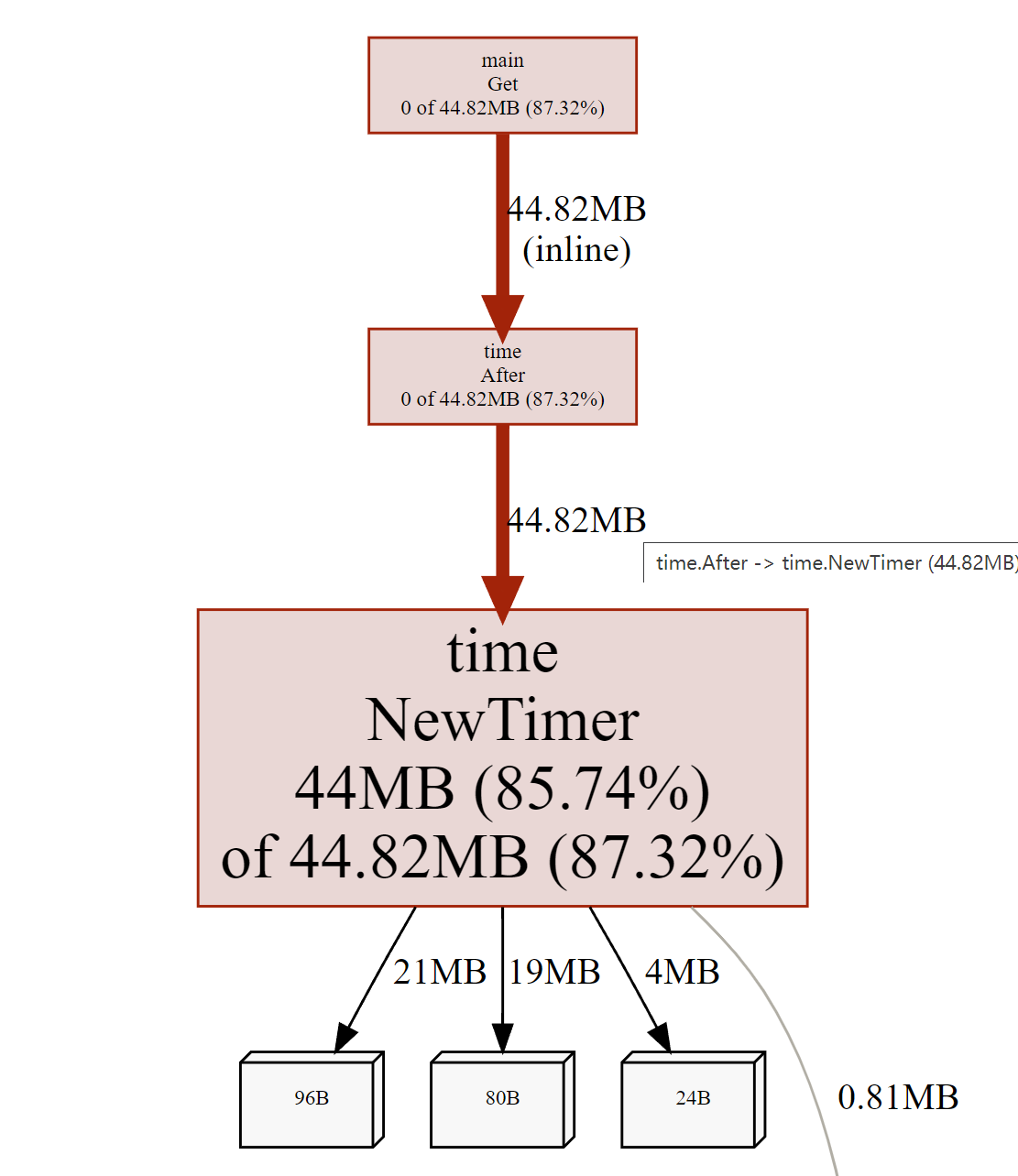``````func Get() {
delay := time.NewTimer(3 * time.Minute)

defer delay.Stop()

for {
delay.Reset(3 * time.Minute)

select {
case v := <- chs:
fmt.Printf("print:%v\n", v)
case <- delay.C:
fmt.Printf("time.After:%v", time.Now().Unix())
}
}
}``````

## HTTP body忘记 Close 导致的泄露

``````type handler struct {
client http.Client
url    string
}

func (h handler) getBody() (string, error) {
resp, err := h.client.Get(h.url)
if err != nil {
return "", err
}

if err != nil {
return "", err
}

return string(body), nil
}``````

``````defer func() {
err := resp.Body.Close()
if err != nil {
log.Printf("failed to close response: %v\n", err)
}
}()``````

## Cache line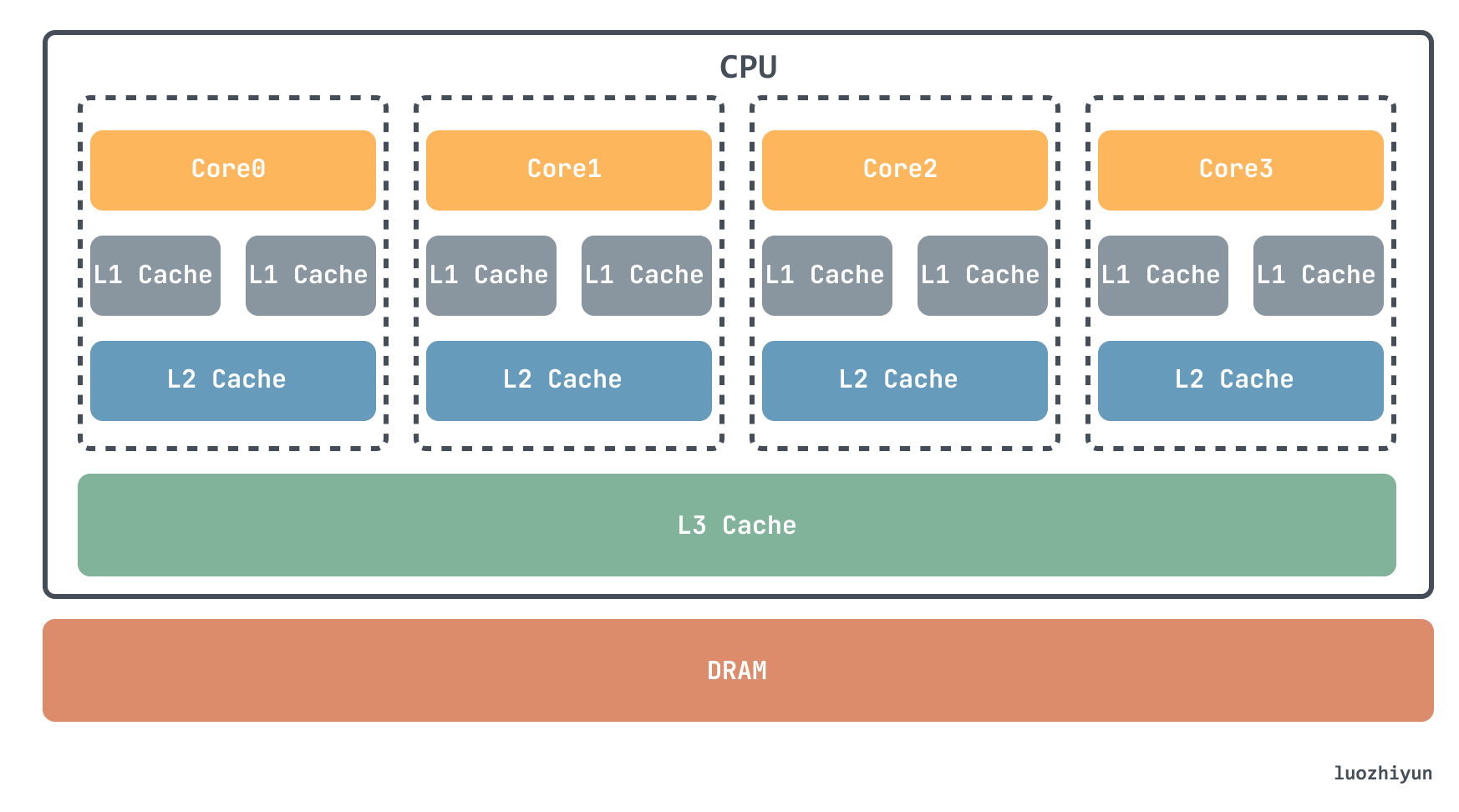cacheline 大小通常有 32 bit，64 bit， 128 bit。拿我电脑的 64 bit 举例：

``````cat /sys/devices/system/cpu/cpu1/cache/index0/coherency_line_size
64``````

``````func sum2(s []int64) int64 {
var total int64
for i := 0; i < len(s); i += 2 {
total += s[i]
}
}

func sum8(s []int64) int64 {
var total int64
for i := 0; i < len(s); i += 8 {
total += s[i]
}
}``````

``````type Foo struct {
a int64
b int64
}

func sumFoo(foos []Foo) int64 {
var total int64
for i := 0; i < len(foos); i++ {
total += foos[i].a
}
}``````

Foo 里面包含了两个字段 a 和 b， sumFoo 会遍历 Foo slice 将所有 a 字段加起来返回。

``````type Bar struct {
a []int64
b []int64
}

func sumBar(bar Bar) int64 {
var total int64
for i := 0; i < len(bar.a); i++ {
total += bar.a[i]
}
}``````

Bar 里面是包含了 a，b 两个 slice，sumBar 会将 Bar 里面的 a 的元素和相加返回。我们同样用两个benchmark 测试一下：

``````func Benchmark_sumBar(b *testing.B) {
s := Bar{
a: make([]int64, 16),
b: make([]int64, 16),
}

b.RunParallel(func(pb *testing.PB) {
for pb.Next() {
sumBar(s)
}
})
}

func Benchmark_sumFoo(b *testing.B) {
s := make([]Foo, 16)

b.RunParallel(func(pb *testing.PB) {
for pb.Next() {
sumFoo(s)
}
})
}``````

``````# go test -gcflags "-N -l" -bench .
Benchmark_sumBar-16     249029368                4.855 ns/op
Benchmark_sumFoo-16     238571205                5.056 ns/op``````

sumBar 会比 sumFoo 快一点的。这是因为对于 sumFoo 来说要读完整个数据才行，而对于 sumBar 来说只需要读前16 bytes 读入到 cache line：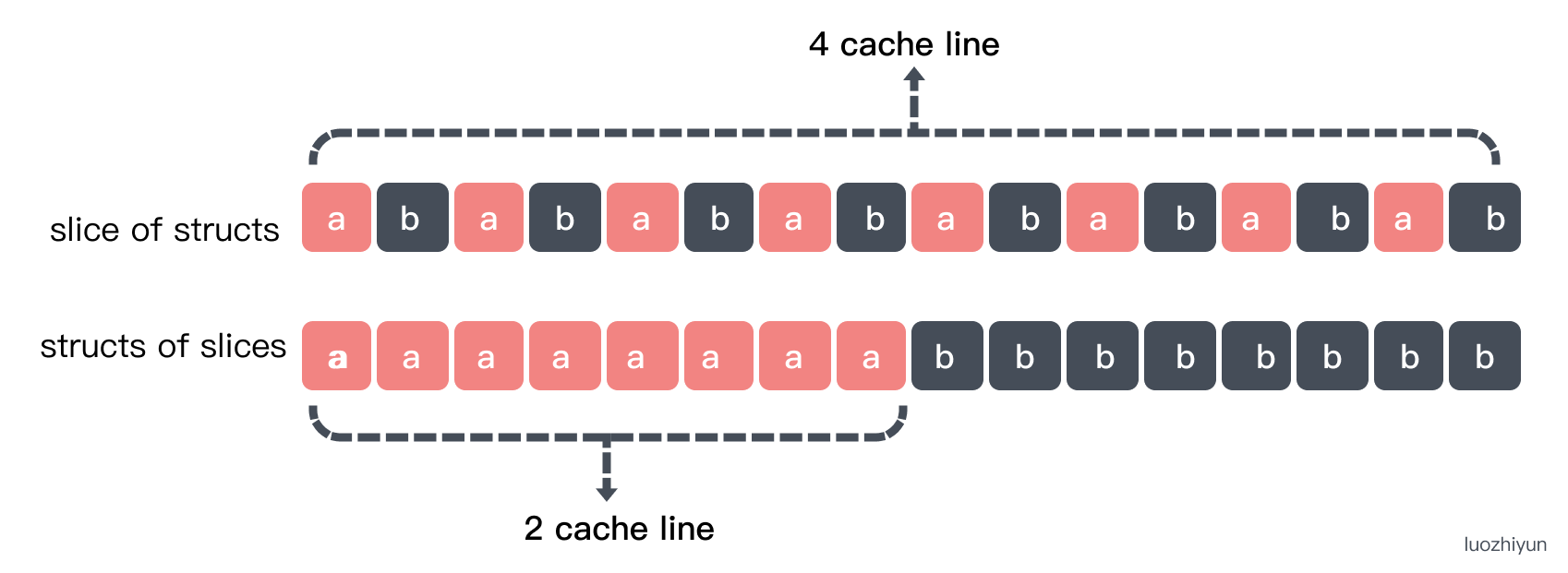## 关于 False Sharing 造成的性能问题

False Sharing 是由于多线程对于同一片内存进行并行读写操作的时候会造成内存缓存失效，而反复将数据载入缓存所造成的性能问题。

CPU 核心里面的 cache 变成无效之后就不能使用了，需要重新加载，因为不同级别的缓存的速度是差异很大的，所以这其实性能影响还蛮大的，我们写个测试看看。

``````type MyAtomic interface {
IncreaseAllEles()
}

a   uint64
_p1 uint64
b   uint64
_p2 uint64
c   uint64
_p3 uint64
}

}

a uint64
b uint64
c uint64
}

}``````

``````func testAtomicIncrease(myatomic MyAtomic) {
paraNum := 1000
var wg sync.WaitGroup
for i := 0; i < paraNum; i++ {
go func() {
for j := 0; j < addTimes; j++ {
myatomic.IncreaseAllEles()
}
wg.Done()
}()
}
wg.Wait()

}
b.ResetTimer()
testAtomicIncrease(myatomic)
}

b.ResetTimer()
testAtomicIncrease(myatomic)
}``````

``````BenchmarkNoPad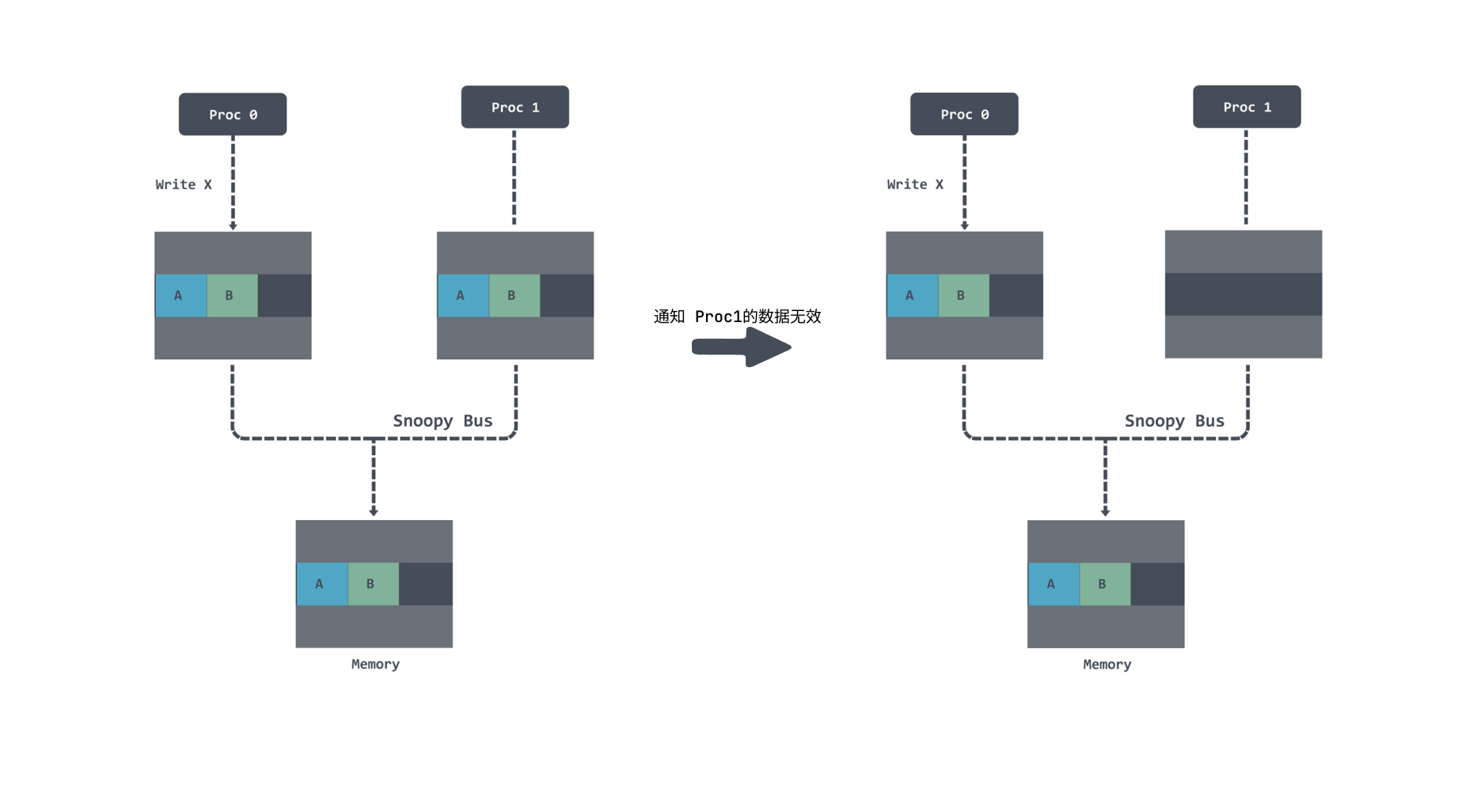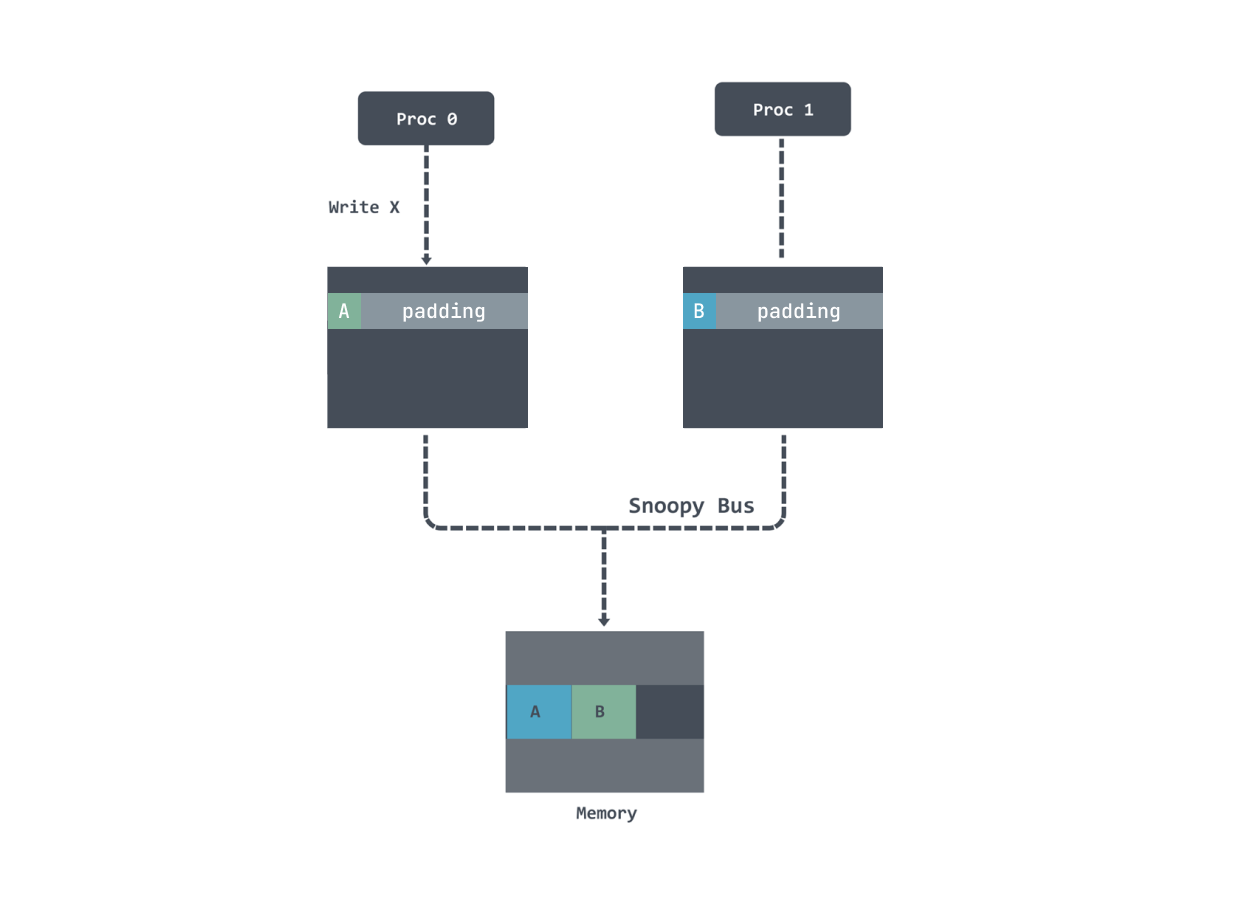## 内存对齐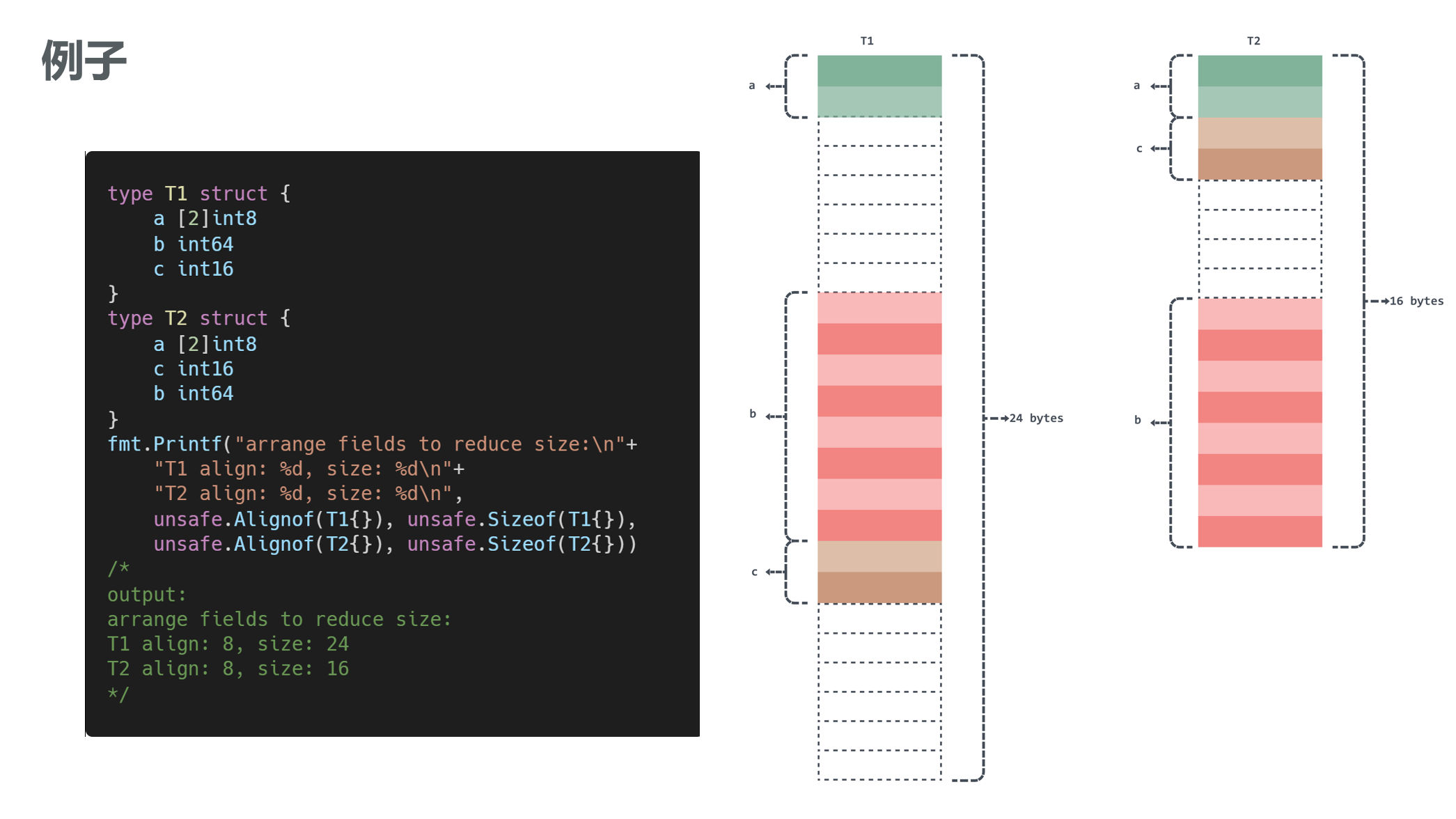``````type M struct {
m int64
x struct{}
}

type N struct {
x struct{}
n int64
}

func main() {
m := M{}
n := N{}
fmt.Printf("as final field size:%d\nnot as final field size:%d\n", unsafe.Sizeof(m), unsafe.Sizeof(n))
}``````

``````as final field size:16
not as final field size:8``````

``````\$ go install golang.org/x/tools/go/analysis/passes/fieldalignment/cmd/fieldalignment@latest

\$ fieldalignment -fix .\main\my.go
main\my.go:13:9: struct of size 24 could be 16``````

## 逃逸分析

Go是通过在编译器里做逃逸分析（escape analysis）来决定一个对象放栈上还是放堆上，不逃逸的对象放栈上，可能逃逸的放堆上。对于 Go 来说，我们可以通过下面指令来看变量是否逃逸：

``go run -gcflags '-m -l' main.go``
• `-m` 会打印出逃逸分析的优化策略，实际上最多总共可以用 4 个 `-m`，但是信息量较大，一般用 1 个就可以了。
• `-l` 会禁用函数内联，在这里禁用掉`内联`能更好的观察逃逸情况，减少干扰。

### 指针逃逸

``````type Demo struct {
name string
}

func createDemo(name string) *Demo {
d := new(Demo) // 局部变量 d 逃逸到堆
d.name = name
return d
}

func main() {
demo := createDemo("demo")
fmt.Println(demo)
}``````

`````` go run -gcflags '-m -l'  .\main\main.go
# command-line-arguments
main\main.go:12:17: leaking param: name
main\main.go:13:10: new(Demo) escapes to heap
main\main.go:20:13: ... argument does not escape
&{demo}``````

### interface{}/any 动态类型逃逸

``````func createDemo(name string) any {
d := new(Demo) // 局部变量 d 逃逸到堆
d.name = name
return d
}``````

### 切片长度或容量没指定逃逸

``````func main() {
number := 10
s1 := make([]int, 0, number)
for i := 0; i < number; i++ {
s1 = append(s1, i)
}
s2 := make([]int, 0, 10)
for i := 0; i < 10; i++ {
s2 = append(s2, i)
}
}``````

`````` go run -gcflags '-m -l'  main.go

./main.go:65:12: make([]int, 0, number) escapes to heap
./main.go:69:12: make([]int, 0, 10) does not escape``````

### 闭包

``````func Increase() func() int {
n := 0
return func() int {
n++
return n
}
}

func main() {
in := Increase()
fmt.Println(in()) // 1
fmt.Println(in()) // 2
}``````

`````` go run -gcflags '-m -l'  main.go

./main.go:64:5: moved to heap: n
./main.go:65:12: func literal escapes to heap``````

## byte slice 和 string 的转换优化

``````// toBytes performs unholy acts to avoid allocations
func toBytes(s string) []byte {
return *(*[]byte)(unsafe.Pointer(&s))
}
// toString performs unholy acts to avoid allocations
func toString(b []byte) string {
return *(*string)(unsafe.Pointer(&b))
}``````

• func Slice(ptr *ArbitraryType, len IntegerType) []ArbitraryType: 返回一个Slice,它的底层数组自ptr开始，长度和容量都是len
• func SliceData(slice []ArbitraryType) *ArbitraryType：返回一个指针，指向底层的数组
• func String(ptr *byte, len IntegerType) string： 生成一个字符串，底层的数组开始自ptr, 长度是len
• func StringData(str string) *byte: 返回字符串底层的数组

## 容器中的 GOMAXPROCS

``````import _ "go.uber.org/automaxprocs"

func main() {
}``````

## Reference

https://go.dev/ref/mem

https://colobu.com/2019/01/24/cacheline-affects-performance-in-go/

https://teivah.medium.com/go-and-cpu-caches-af5d32cc5592

https://geektutu.com/post/hpg-escape-analysis.html

https://github.com/uber-go/automaxprocs

https://gfw.go101.org/article/unsafe.html